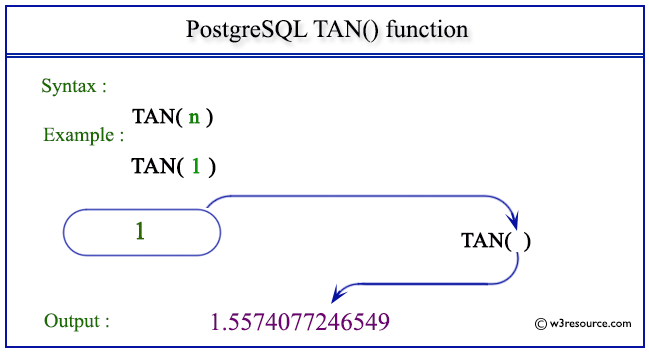# PostgreSQL TAN() function

## TAN() function

The PostgreSQL tan() function is used to return the tangent of a given argument.

Syntax:

```tan(a)
```

PostgreSQL Version: 9.3

Pictorial presentation of PostgreSQL TAN() functionExample 1: PostgreSQL TAN() function

Code:

``````SELECT TAN(0) AS "Tan (0)";
```
```

Sample Output:

``` Tan (0)
---------
0
(1 row)
```

Example 2: PostgreSQL TAN() function

Code:

``````SELECT TAN(1) AS "Tan (1)";
```
```

Sample Output:

```     Tan (1)
-----------------
1.5574077246549
(1 row)
```

Example 3: PostgreSQL TAN() function

Code:

``````SELECT TAN(-1) AS "Tan (-1)";
```
```

Sample Output:

```     Tan (-1)
------------------
-1.5574077246549
(1 row)
```

Previous: SQRT function
Next: TRUNC function

﻿